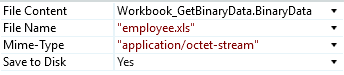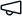Give us feedback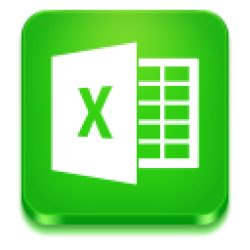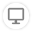# Excel Package

Stable version 2.0.0 (Compatible with OutSystems 11)
Other versions available for 10 and Older
Published on 17 June 2020 by
5.0
(6 ratings)# Excel Package

Documentation
2.0.0

With this component, you can create advanced Excel 2007/2010 spreadsheets on the server using EPPlus .NET library.

This component provides several actions that allow you to use some of the functionalities of Excel:

• Cell_CalculateByIndex -  This action allows you to calculate the formula of a cell, defined by its index. It has as an input parament the Worksheet Object, the row, and the column.

• Cell_CalculateByName - With this action, you can calculate the formula of a cell, defined by its name. Has an input parament the Worksheet Object and the cell name.

• Cell_FormatRange - Using this action you can apply a format to a range of cells. Has as input parameter the Worksheet Object, the Row and Column Start, the Row and Column end and the format you want to apply.

• Cell_ReadByIndex - This action reads the value of the cell, defined by its index. Has as input parameter the WorkSheet Object, the Row and Column, and a ReadText (Boolean) if set to true always reads the cell value as text.

• Cell_ReadByName - This action has the purpose of the action above but instead of giving an index, reads the cell by its name.

• Cell_SetFormulaByIndex - With this action you can write a formula to a cell by its index. Has as input parameters the Worksheet Object, the Row and Column and the formula you want to apply.

• Cell_SetFormulaByName - Has the same purpose of the action above but instead of a given index this action uses the name of the cell

• Cell_WriteByIndex - Writes a converted value to a cell, defined by its index. Has as input parameters the Worksheet Object, the Row and Column, the CellValue and the Cell Type.

• Cell_WriteByName - This action has the same purpose of the action above but instead of giving an index writes on the cell according to a given cell name.

• Cell_WriteByIndexWithFormat - Write a converted value to a cell, defined by its index. Has as input parameters the Worksheet Object, the Row and Column, the CellValue, the CellType and the CellFormat that you want.

• Cell_WriteByNameWithFormat - Has the same purpose of the action above but instead of receiving an index receives a name.

• Cell_WriteColumnRange - This action writes a dataset to a range of column cells. Has as input parameters the Worksheet Object, the Row, The Column Start, the Value List, and the CellType.

• Cell_WriteColumnRangeWithFormat - This action is similar to the action above but with this action, you can add a format to the target cells. Has the same input parameters of the action above plus the format.

• Cell_WriteImageByIndex - This action writes an image on a cell, defined by its index. Has as input parameters the WorksheetObject, the Row and Column, the ImageName and the Image.

• Cell_WriteImageByName - This action has the same purpose of the action above but instead of receiving an index it receives the name of the cell

• Cell_WriteRange - Writes a dataset to a range of cells. Has as input parameters the Worksheet, the Row and ColumnStart and the Dataset.

• Cell_WriteRangeWithFormat - Has the same behavior of the action above but you can add a format to the target cells.

• Column_Hide - Hides/Show a column by index. Has as input parameters the Worksheet Object, the Column and Hidden that is a boolean where you can select if to hide the column or not

• Workbook_AddCopyWorksheet - This action adds a copy of a worksheet. Has as input parameter the Workbook, the WorksheetName, the WorksheetToCopy.

• WorkBook_AddName - Add a worksheet to work on by its name. Has as input parameters the Workbook and the Worksheet name.

• Worbook_Calculate - Calculates all formulas in the Workbook. Has as input parameter the Workbook.

• Workbook_Close -  Closes the excel workbook. Has as input parameter the Workbook.

• WorkBook_Create - Creates a new excel workbook. Has as input parameters the number of sheets and the FirstSheetName

• Workbook_GetBinaryData - This action returns the excel workbook as binary data. Has as input parameters the Workbook

• Workbook_GetProperties - Returns a list of the Worksheets contained in the Workbook. Has as input parameter the Workbook.

• Workbook_Open - Opens an existing workbook for editing. Has an input parameter the Filename.

• Workbook_Open_BinaryData - Opens an existing workbook for editing and keep it in memory. Has as input parameters the binary data

• Worksheet_AddName - This action creates a defined “Name” (a word or string of characters in Excel that represents a cell, range of cells, formula or constant value) in excel, starting in the RowStart/ColumnStart cell. Has as input parameters the Worksheet, the Name, the Dataset, and the Row and Column Start.

• Worksheet_Calculate - This action calculates all formulas in one worksheet. Has as input parameter the worksheet.

• WorkSheet_DeleteByIndex - Deletes a worksheet in a workbook by index. Has as input parameters the Workbook and the index.

• Worksheet_DeleteByName - Same behavior of the action above but instead of a given index it receives a name

• Worksheet_GetImages - This action returns all images from a worksheet. Has as input parameter the WorkSheet.

• Worksheet_GetName - This action returns the name of the worksheet. Has as input parameter the Worksheet.

• Worksheet_GetProperties - This action returns the following properties of the Worksheet:

• Name

• Index

• Tab Color

• A (Alpha)

• R (Red)

• G (Green)

• B (Blue)

• Name

• IsSystemColor

• IsknownColor

• IsNamedColor

• Dimension

• Rows

• Columns

If there is no data in the sheet, the rows and columns will return 0, and the address property will be undefined

• Worksheet_Protect - This action protects a worksheet with a password. Has as input parameter the Worksheet and the password

• Worksheet_Rename - This action allows you to rename a worksheet.

• Worksheet_SelectByIndex - This action selects a worksheet by its index. Has an input parameter the Workbook and the Worksheet number.

• Worksheet_SelectByName - This action has the same behavior of the action above but instead of an index it receives a name.

How to use

In order to explain the use of this component, let's put together an example. We are going to use the SampleDB provided by OutSystems. The entity sample is going to be the Employee and the result will be an Excel spreadsheet with information about the employees.

Note: You need to create an Excel spreadsheet and put it in the Resources folder under the Data Tab.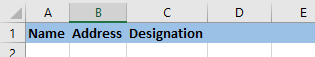Step1 - Create a Web Application and import PackageExcel and SampleData.

Step 2 - Add a Screen and name it SampleScreen.

Step 3 - Add a button to this screen and create a Screen Action to serve as the destination of this button. Also had a local variable with the name of RowCount, of the Type Integer and set the default value to 2.

Step 4 - Open the recently created screen action.

a) First, drag an Aggregate and set the source to the Sample_Employee entity.

b) Below this aggregate, drag the action Workbook_Open_BinaryData. The binary data is the Excel that you put on the Resources Folder.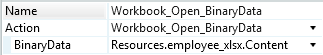Step 5 - Next, drag the Worksheet_SelectByIndex action.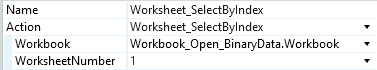Step 6 - You will need a For each where the Record List is the list returned by the aggregate created before.

Step 7 - On the left of the for each, drag a Cell_WriteByIndex. This action is going to be used three times. One for each column. (Name, Address, JobPosition).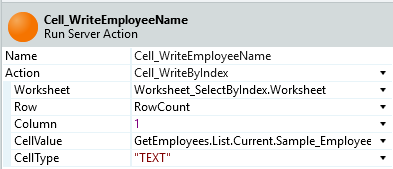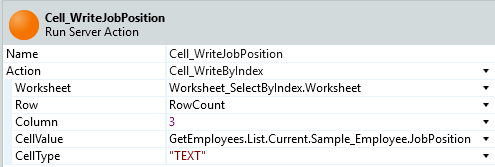Note: In the first image, the CellValue is the FirstName + LastName

Step 8 - Next, drag an Assign and increase the RowCount variable in one.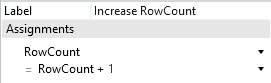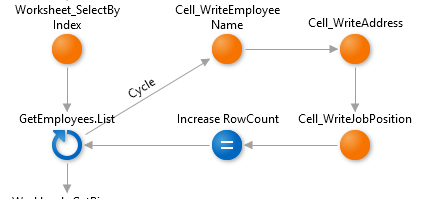Below the For Each, drag the Workbook_GetBinaryData action to obtain the workbook.

Step 9 - After that, you just need to download it. Drag a Download statement and put it between the Workbook_GetBinaryData action and the End.